Change List for this Rule

General Order 95

Appendix F

Typical Problems

Part II

It is the object of this problem to indicate the construction requirements for a typical deadend structure, since the longitudinal stresses imposed upon such a structure differ substantially from those on a pole on which the conductors supported are normally balanced. The deadend structure considered herein is assumed to support an 11,000 volt circuit, a 4,000 volt circuit and two secondary circuits. It is also assumed that the deadend pole takes Grade A construction by virtue of its location.

The deadend structure diagram and dimensions are shown on Page F22 . The primary data chosen for this structure are as follows:

Data For Deadend Structure

Supply Conductors

 11 kV circuit 3 #0 AWG Stranded, harddrawn copper 4 kV circuit 4 #2 AWG Stranded, harddrawn copper 120/240 volt circuit 3 #4 AWG Solid, harddrawn copper 120/240 volt circuit 3 #2 AWG Stranded, harddrawn copper

Insulators - Strain Type (to conform to Rule 49.5 ).

Conductor fastenings (to meet the safety factor of Table 4 , Rule 44.1 )

Crossarms:

 11 kV circuit Douglas fir 43/4 x 53/4 x 80 4 kV circuit Douglas fir 43/4 x 43/4 x 78 Secondary circuits Douglas fir 43/4 x 43/4 x 70

Crossarm braces (to conform to Rule 48.2 and 49.8 )

Pole - western red cedar.

Pole dimensions: 55 in length; 25 top circumference; 50 ground line circumference (ground line diameter 15.9).

Construction Requirements

1.    Conductor Tensions

It is assumed that the conductors are strung with the minimum sags specified in sag curves of Appendix C , hence the tension values at 60_F and no wind (normal tensions) are 35% of the ultimate tensions shown in Table 18 . These tensions for each of the conductor sizes and corresponding tensions at maximum loading (25°F and wind of 8 pounds) are as follows, where span length is 250 feet:

 TensionPounds 35% of Ultimate At Maximum Loading #O AWG Stranded, harddrawn copper 1,664 2,125 #2 AWG Stranded, harddrawn copper 1,065 1,360 #4 AWG Solid, harddrawn copper 690 890

2.    Crossarms

Spacings assumed are shown on the pole framing diagram on Page F22 . Double crossarms of Douglas fir, dense, are employed for each of the four different circuits.

Computations of the fiber stresses imposed upon the various crossarms by the unbalanced wire loads of conductors in the physical configuration shown on the diagram are made in accordance with the method outlined in Part 1 to show these stresses under the conditions of longtime loading and maximum loading. Furthermore, double crossarm construction of this type with separation maintained by space bolts is assumed to have a horizontal strength equivalent to 130% of the sum of the strengths of two single crossarms acting independently. The stresses computed in this manner are:

 Fiber Stress - lbs per Sq. In. LongTime Maximum Loading Loading Top crossarms 1,412 1,804 Second Crossarms 1,598 2,040 Third crossarms 932 1,202 Fourth crossarms 1,438 1,811

Since a factor of safety of 2 permits a maximum stress of modulus of rupture in bending of 1,732 lbs per square inch.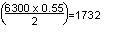under the conditions of long-time loading (60o F and no Wind) and 3150 lbs per square inch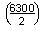at maximum loading see Table 5 the crossarm chosen are satisfactory.

3.    Pole (See Page F22 )

Rule 44 provides that poles supporting unbalanced longitudinal loads in Grade A construction shall have a safety factor of 4 against such loads. Rule 47 specifies that guys used to support unbalanced longitudinal loads shall have a safety factor of 2 for all grades of construction (Where guys are used they must take the entire load with the designated safety factor, the pole being considered merely as a strut).

Using the values given above for tensions at maximum loading, the following moments due to dead ending the conductors are obtained:

 3 x 2,125 x 47.3 = 301,500 poundfeet 4 x 1,360 x 38.3 = 208,400 poundfeet 3 x 890 x 30.3 = 80,900 poundfeet 3 x 1,360 x 25.3 = 103,200 poundfeet Total Moments = 694,000 poundfeet

The total deadend stress, using the tension values for maximum loading given above, will be:

 3 x 2,125 = 6,380 pounds 4 x 1,360 = 5,440 pounds 3 x 890 = 2,670 pounds 3 x 1,360 = 4,080 pounds Total = 18,570 pounds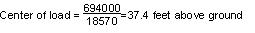The tension of a single guy with a lead to height ratio of 1 to 1 (assumed) and a safety factor of 2 would be: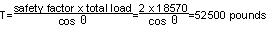A stranded guy attached at the center of load could be used provided the allowable fiber stress of the pole is not exceeded. The stress due to guying at this point would be as follows:

The center of load (37.4 above ground) would be

9.9 ft. (118.8) below the top conductors (11 kV) and

0.9 ft. ( 10.8) below the second crossarm (4 kV)

The fiber stress in the pole at the center of load due to the tension in the conductors above the center of load is computed as follows:

 Bending moment 3 x 2,125 x 118.8 = 757,400 poundinches 4 x 1,360 x 10.8 = 58,800 poundinches Total moment = 816,200 poundinches

The section modulus of a solid circular section is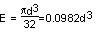The diameter of the pole at the center of load is d = 9.7inches

Then, E = 0.0982 x (9.7)3 = 89.6 3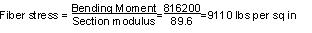Since a pole in Grade A construction must have a safety factor of 4, the allowable value of fiber stress would be 5,600/4 = 1,400 pounds per square inch; therefore, the pole cannot be guyed by a single guy but can be guyed as illustrated on Page F22.

Note:      Revised February 5, 2014 by Decision No. 14-02-015.